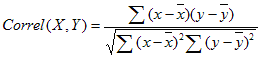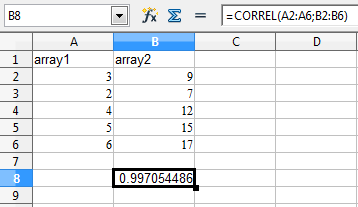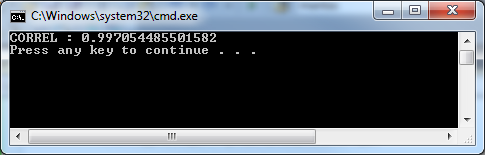# C#: How to get correlation coefficient of two arrays Correl()

This simple example shows you how to get the correlation coefficient of two arrays.  Microsoft Excel and OpenOffice Calc has a function for this called CORREL() :```//Two arrays double[] array1 = { 3, 2, 4, 5, 6 }; double[] array2 = { 9, 7, 12, 15, 17 };   double[] array_xy = new double[array1.Length]; double[] array_xp2 = new double[array1.Length]; double[] array_yp2 = new double[array1.Length]; for (int i = 0; i &lt; array1.Length; i++) array_xy[i] = array1[i] * array2[i]; for (int i = 0; i &lt; array1.Length; i++) array_xp2[i] = Math.Pow(array1[i], 2.0); for (int i = 0; i &lt; array1.Length; i++) array_yp2[i] = Math.Pow(array2[i], 2.0); double sum_x = 0; double sum_y = 0; foreach (double n in array1) sum_x += n; foreach (double n in array2) sum_y += n; double sum_xy = 0; foreach (double n in array_xy) sum_xy += n; double sum_xpow2 = 0; foreach (double n in array_xp2) sum_xpow2 += n; double sum_ypow2 = 0; foreach (double n in array_yp2) sum_ypow2 += n; double Ex2 = Math.Pow(sum_x, 2.00); double Ey2 = Math.Pow(sum_y, 2.00);   double Correl = (array1.Length * sum_xy - sum_x * sum_y) / Math.Sqrt((array1.Length * sum_xpow2 - Ex2) * (array1.Length * sum_ypow2 - Ey2));   Console.WriteLine("CORREL : "+ Correl);```## 2 thoughts on “C#: How to get correlation coefficient of two arrays Correl()”

1. Alex

Your code isn’t working properly, maybe you made mistake in this code? Now i’m trying to use your code, but unfortunately all calculation are wrong….

2. S

private static double CalculateCorrelationCoeff(double[] SR, double[] IdxR)
{
double output = 0.0d;

double[] RelationStk = new double[SR.Length];
double[] VarianceStk = new double[SR.Length];
double[] VarianceIdx = new double[SR.Length];

double SRMean = SR.Average();
double IdxMean = IdxR.Average();

for (int i = 0; i <= SR.Length – 1; i++)
{
RelationStk[i] = (SR[i] – SRMean) * (IdxR[i] – IdxMean);
VarianceStk[i] = (SR[i] – SRMean) * (SR[i] – SRMean);
VarianceIdx[i] = (IdxR[i] – IdxMean) * (IdxR[i] – IdxMean);
}

double coVarianceStk = RelationStk.Sum() / VarianceStk.Length;
double stDevStk = Math.Sqrt((VarianceStk.Sum()) / VarianceStk.Length);
double stDevIdx = Math.Sqrt((VarianceIdx.Sum()) / VarianceStk.Length);

output = coVarianceStk / (stDevStk * stDevIdx);

return output;
}